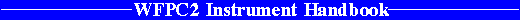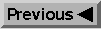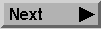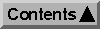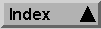System Throughput and SNR / Exposure Time Estimation

# 6.7 Sample SNR Calculations

Below we give further examples of SNR calculations. The Appendix also gives tables of SNR values for a wide range of representative cases.

## 6.7.1 Point Sources

### Simple Star, Manual Calculation, PSF Fitting

We begin with the simple example of V=20 star of spectral class G0. We want to observe with the PC using filter F555W. The star is somewhere near the ecliptic pole. We want to know the SNR for a 1200s CR-SPLIT exposure. Default ATD-GAIN=7 is used. We plan to use PSF fitting to analyze the data.

First we estimate the count rate for our target. Consulting Equation 6.2, Table 6.1, and Table 6.2 we have: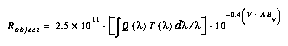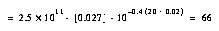in units of e- s-1. Next we fill out Equation 6.6. To keep things simple we just use values from Table 6.6, and get the sky count rate from Table 6.4. There is no background light (i.e. no superposed galaxy), so Pbackground=0. The exposure time t=600 for each exposure of the CR-SPLIT: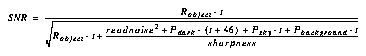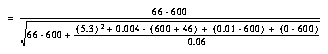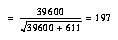The SNR for the total 1200s exposure, i.e. both halves of the CR-SPLIT, would simply be: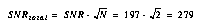At these high SNR levels, it is likely that flat fielding would limit the photometric accuracy, rather than the noise. If we have a look at the terms in the SNR equation, we can see that the Poisson noise dominates; the term containing the sharpness and background noise sources is unimportant.

Just for fun, let us see what happens if we keep everything the same, but give the target V=25. Now we have Robject=0.66 e- s-1, and: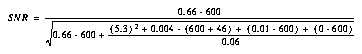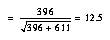We see that now the term with the background noise (in particular, the read noise) limits the SNR. For the full 1200s exposure the SNRtotal=17.6.

### Simple Star, Manual Calculation, Aperture Photometry

What if we now want to observe this same V=25 star, but we plan to reduce the data by measuring counts in a 0.5" radius aperture? We now use Equation 6.7 instead, consult Table 6.7 for the encircled energy f(r), and note that 0.5" corresponds to r=11.6 PC pixels: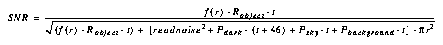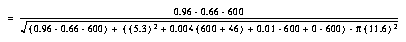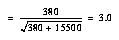Apparently using aperture photometry with a 0.5" radius aperture reduces the SNR by a factor ~4 as compared to PSF fitting, for this background limited case.

### Simple Star, SNR Tables, PSF Fitting

We now repeat the first calculation above for the V=20 star using the SNR tables in Appendix B. We look up the G0 spectral class and F555W filter (5500Å) in Table B.1, and obtain ABnu=0.02. For the V=20 star, we thus have V+ABnu=20.02. We look at Figure B.10 on page 234 and find this value on the horizontal axis. We locate exposure time 600s (one-half of the total 1200s CR-SPLIT exposure), and find SNR~200. For the total 1200s exposure the SNR would be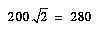.

### Simple Star, On-Line Calculator, PSF Fitting

The above calculation for a V=20 GO star may also be performed using the WFPC2 Exposure Time Calculator

#### Figure 6.2: Sample Fill-out Form for WFPC2 On-Line Exposure Time Calculator.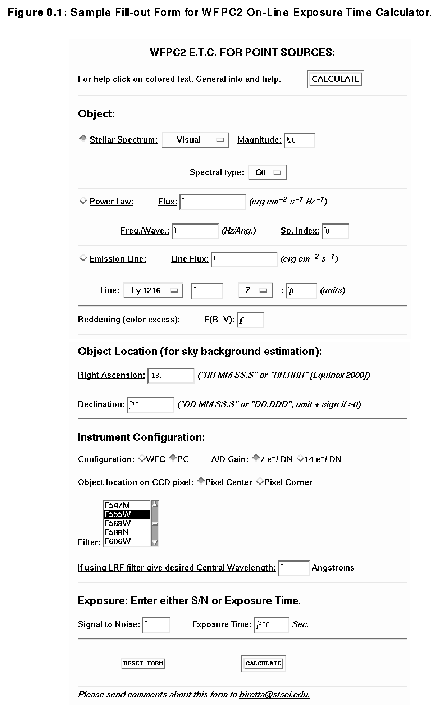program, which is available on the WFPC2 WWW pages at:

``` `````` http://www.stsci.edu/ftp/instrument_news/WFPC2/wfpc2_top.html```

To use this program, access the above address with NCSA Mosaic, Netscape, or a similar program. Once in the WFPC2 area, select the "Software Tools" page, and then the "ETC" page. For the first example above, choose the "Point Source" form and complete it as shown in Figure 6.2 for the 600s sub-exposure. Then clicks the "calculate" button and after a few seconds the result is displayed (Figure 6.3). The answer, SNR=198, is comparable to that obtained by the manual calculation above for the 600s sub-exposure (SNR=197). Alternatively, one can input the total exposure time (1200s), and then use the result farther down the output page for "No. Sub-Exposures = 2" (see Figure 6.4), thereby obtaining SNR=277 for the total 1200s CR-SPLIT exposure.

#### Figure 6.3: Sample Results from WFPC2 On-Line Exposure Time Calculator.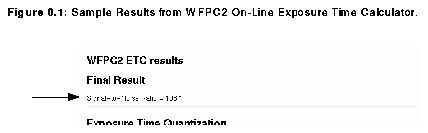#### Figure 6.4: Sample Results on CR-SPLITing from WFPC2 On-Line Exposure Time Calculator Results Page.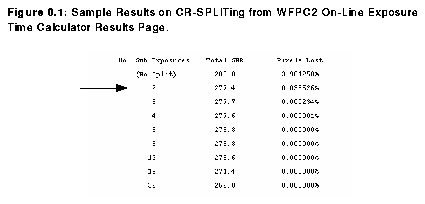### Star Superposed on Galaxy, Manual Calculation

We now consider a B=25 point source of spectral class B0, which is superposed on an elliptical galaxy with sigma(V)=22 mag arcsecond-2. We want to compute the SNR obtained from a one-orbit (40 min.) non-CR-SPLIT observation in filter F300W on the WFC. PSF fitting will be used for the photometry.

We begin by computing the total count rate for the target. Using Table 6.2 we see that this target will have V=25.31. From Table 6.1 we obtain the filter efficiency and mean wavelength. Interpolating by mean wavelength in Table 6.2 we obtain ABnu=-0.83 for the B0 star. Using Equation 6.2 we have: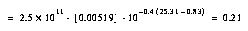in units of e- s-1. Next we consider the background light from the superposed galaxy. We set sigma(V)=22 mag arcsecond-2 in Equation 6.11, and ABnu=3.63 for a gE galaxy at lambda=3000Å (filter F300W) from Table 6.2. Hence the count rate per pixel due to the background light is: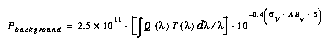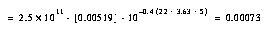For the sky background, we note that Table 6.4 has no entry for F300W, so that the sky must be unimportant. If we wanted to calculate it anyway, as a check, we would use Table 6.3 for the sky brightness, Table 6.2 for the sky's ABnu, and again Equation 6.11. We will assume the target is near the ecliptic pole.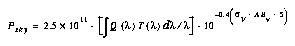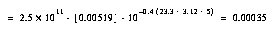For the sharpness function we will use "pixel corner" values (least optimistic choice) from Table 6.5. Using read noise and dark current from Table 6.6, and Equation 6.6 for point source SNR: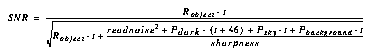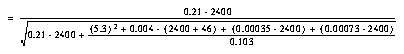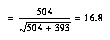for this single exposure. The SNR for multiple 40 min. exposures would be simply 16.8(N1/2), where N is the number of exposures.

### Star Superposed on Galaxy, On-Line Calculator

The above calculation could also be performed with the on-line WFPC2 Exposure Time Calculator. One would select the "Point source + stellar background" form, and complete it as in Figure 6.5,

#### Figure 6.5: Point Source + Stella Background Fill-out Form for WFPC2 On-Line Exposure Time Calculator.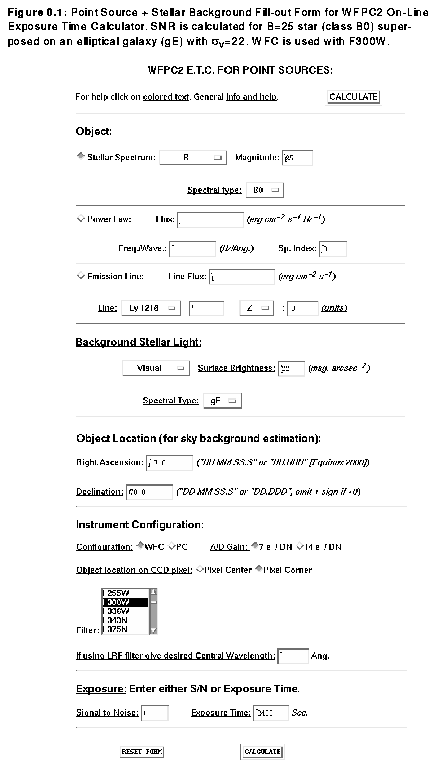and then click on "calculate." Figure 6.6 shows some of the results.

## 6.7.2 Extended Sources

In general, the signal-to-noise level for extended sources can be computed by comparing the expected signal, S, in each pixel, determined from Equations 6.8 through 6.13, to the noise N=(S+B)1/2, where B is the equivalent background, determined in a manner similar to that for point sources. Unlike for point sources, the calculation does not, in a first approximation, involve the sharpness of the point spread function. For example, let us consider the observation of a source with a V surface brightness of 24 mag arcsec-2, assuming the F569W filter F569W, WFC camera, and sky background V=23.3 mag arcsec-2. The signal-to-noise estimate goes as follows. The signal in each WFC pixel is 24.0+5.0 = 29.0 magnitude. By Equation 6.11, the total signal collected from the source in a 3000 second integration is S = 41.8 electrons, neglecting the small AB color correction. The sky signal per pixel is 76.8 electrons. The dark current is ~12 electrons. The total equivalent background is thus B = 76.8+5.32+12 = 116.9 electrons, larger than the signal detected, thus the noise is background-dominated. The noise is N=(S+B)1/2= 12.6 electrons, and the signal-to-noise per pixel expected in this case is 3.3. Similar calculations can be carried out for other filters; for observations in narrow-band filters and in the UV, the sky background signal will usually be unimportant. For very long observations of faint objects, other noise terms, such as flat field uncertainty, and errors in dark (and possibly bias) subtraction, must be considered more carefully.

## 6.7.3 Emission Line Sources

#### Figure 6.6: Sample Output from WFPC2 On-Line Exposure Time Calculator.The signal-to-noise ratio calculation for point-like or extended emission-line sources is similar to that for continuum sources. However, the details of the calculation are different, because of the units used for the line flux, and because the flux is in a narrow line. The integrated filter efficiency is not relevant for the signal calculation; what matters is the total system throughput QT at the wavelength of the line, which can be determined from inspection of the Figures in
section A.2, "Passbands including the System Response", on page 211. For lines near the center of the filter bandpasses the QTmax values from Table 6.1 can be used. The total signal expected for a point source of line strength F, expressed in erg s-1 cm-2, is S=2.28x1012 lambda t QT F, where t is the exposure time in seconds, and lambda the wavelength of the line in Angstroms. Thus, H_a emission at 6563Å, with flux F=10-16 erg s-1 cm-2, observed for 1000 seconds through the F656N filter (total system throughput QT=0.104 from "F656N, F658N, F673N, F675W, F702W, F785LP" on page 217), will produce a total signal of S=155 electrons. The equivalent background per pixel is read-noise dominated: B=1+5.32+4=33, for a background noise of ~6 electrons. The total noise is dominated by photon noise from the signal itself, and the signal-to-noise ratio achieved in this observation is over 10.

If the source is extended, the expected signal per arcsecond must be multiplied by the effective pixel area: 0.0099 arcsec2 for the WF, 0.0021 for the PC. For a line flux of, say, F = 10-15 erg s-1 cm-2 arcsec-2, this corresponds to 15 electrons in 1000 seconds for a WFC pixel. The noise is now dominated by the background, and the single-pixel signal-to-noise ratio is 15/(33 + 15)1/2 ~ 2.1.

### Extended Line Emission Source, Manual Calculation

We now consider a detailed example of a planetary nebula observed on the PC with the F502N filter. The nebula has a diameter of 5" and a total flux F=4x10-13 erg s-1 cm-2 in the [OIII] 5007Å line. We want to estimate the SNR for an 1800s exposure, which will be CR-SPLIT.

First we must estimate the flux per square arcsecond. Using the nebula diameter, the average brightness is Inu = 2.0x10-14 erg s-1 cm-2 arcsec-2. From the plots in section A.2, "Passbands including the System Response", on page 211, we see that QT=0.053. Using Equation 6.10 for the target count rate per pixel: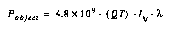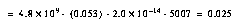Next we estimate the SNR for each 900s sub-exposure using Equation 6.14 and Table 6.9. For this narrow filter the sky background can be ignored. We presume there is no background light from astrophysical sources: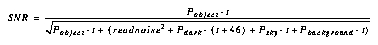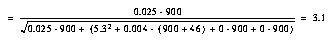Hence SNR=3.1 per pixel for each 900s sub-exposure. The SNR per pixel for the total 1800s is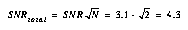The SNR for the entire nebula is this SNR per pixel times the square root of the number of pixels in the image, or ~420. In actuality, uncertainties in the photometric calibration and flat fields, would limit the SNR to ~100.

### Extended Line Emission Source, On-Line Calculator

The above example could be calculated with the "Extended Source" form of the ETC program. The fill-out form would be completed as shown in Figure 6.7.

#### Figure 6.7: Extended Source Form for WFPC2 On-Line Exposure Time Calculator.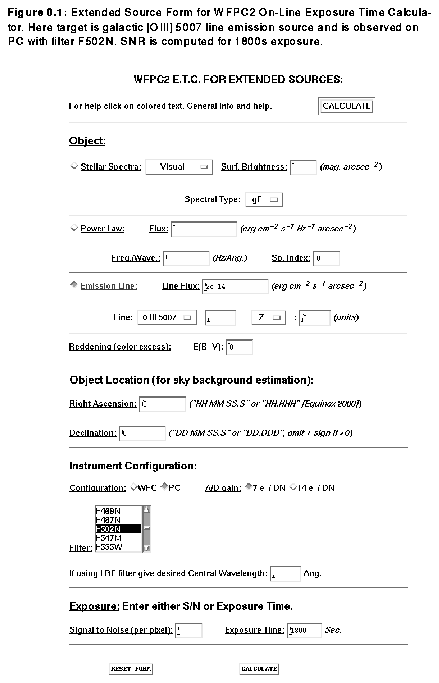We have selected "[OIII] 5007" on the emission line menu, and have left the redshift (z) set to zero. The PC and F502N filter are selected. Note we have entered the exposure time as 1800s. Scrolling down through the output page we find a table of SNR for various CR-SPLITings of the exposure (See Figure 6.8). "No. Sub-Exposures = 2" gives the answer we want, SNR=4.5 per pixel.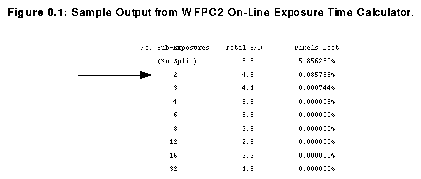### Line Emission Point Source w/ LRF, Manual Calculation

In this example we consider an unresolved source of Ha emission in a galaxy at redshift z=0.22 with flux F=1.5x10-16 erg s-1 cm-2. We want the SNR for a 2400s exposure without CR-SPLITing.

Since the redshift is significant, we cannot observe with the F656N filter. Instead we will use the Linear Ramp Filter (LRF). The observed wavelength will be 8007Å. From Table 3.7 on page 48 we see that this will be observed using the FR868N filter on CCD WF3. Combining the LRF transmission from Figure 3.2 and the "WFPC2 + OTA System Throughput" from Figure 2.4 we estimate QT=0.054. We compute the count rate using Equation 6.4: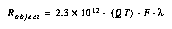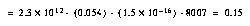To estimate the SNR we use Equation 6.6, which assumes that PSF fitting will be used to analyze the image. Since the filter is narrow, we will ignore the sky emission. We use Table 6.6 for the WFC sharpness and also the read noise.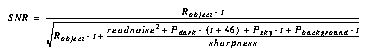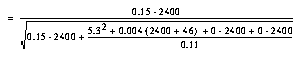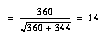### Line Emission Point Source w/ LRF, On-Line Calculator

The above calculation
can be performed using the ETC program. The "Point Source" form is used. "Emission Line" source and the "H 6563" line are selected; the redshift is set to 0.22. The program will automatically choose between PC and WFC, depending on the LRF setting. The least optimistic case of placing the object on a "pixel corner" is selected. The filter "LRF" is selected from the filter menu, and 8007Å is given for the central wavelength. The exposure time is specified as 2400s. (See Figure 6.9 for example of completed form.)

#### Figure 6.9: Point Source for WFPC2 On-Line Exposure Time Calculator.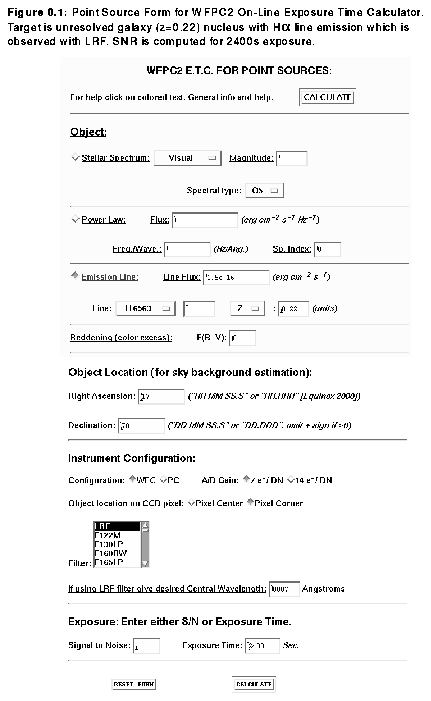The result is SNR=13.1 for the un-split 2400s exposure (Figure 6.10), which is comparable to the manual calculation of SNR=14.

#### Figure 6.10: Sample Output for WFPC2 On-Line Exposure Time Calculator.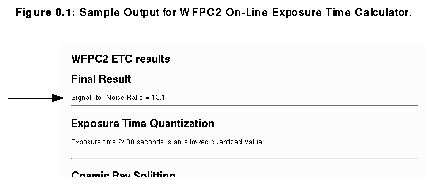6.7.1 - Point Sources
Simple Star, Manual Calculation, PSF Fitting
Simple Star, Manual Calculation, Aperture Photometry
Simple Star, SNR Tables, PSF Fitting
Simple Star, On-Line Calculator, PSF Fitting
Star Superposed on Galaxy, Manual Calculation
Star Superposed on Galaxy, On-Line Calculator
6.7.2 - Extended Sources
6.7.3 - Emission Line Sources
Extended Line Emission Source, Manual Calculation
Extended Line Emission Source, On-Line Calculator
Line Emission Point Source w/ LRF, Manual Calculation
In this example we consider an unresolved source of Ha emission in a galaxy at redshift z=0.22 with flux F=1.5x10-16 erg s-1 cm-2. We want the SNR for a 2400s exposure without CR-SPLITing.
which is for an un-split 2400s exposure. The Poisson noise and background noises contribute nearly equally. For three such exposures over three orbits
Line Emission Point Source w/ LRF, On-Line Calculator